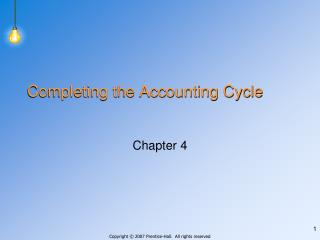# Completing the Accounting Cycle - PowerPoint PPT PresentationDownload PresentationCompleting the Accounting Cycle

Completing the Accounting Cycle
Download Presentation## Completing the Accounting Cycle

- - - - - - - - - - - - - - - - - - - - - - - - - - - E N D - - - - - - - - - - - - - - - - - - - - - - - - - - -
##### Presentation Transcript

1. Completing the Accounting Cycle Chapter 4

2. The worksheet helps accountants with all of the following except: • Post to the accounts • Prepare financial statements • Close the accounts • Make adjusting entries

3. Answer: 1 The worksheet is a tool that helps accountants organize the end-of-year activities – preparing adjusting and closing entries and the financial statements.

4. On the work sheet, in the balance sheet columns, if the total credits are \$600 and total debits are \$200, then • An error has been made • Net loss is \$400 • Total assets are \$400 • Net income is \$400

5. Answer: 2 The difference between the debit and credit columns is the amount of net income or loss, which is used to balance the columns. In this case, \$400 is needed in the debit column to balance them. A debit indicates that capital is decreasing.

6. Granite Company had revenues of \$600 and expenses of \$200 during the year. The owner’s beginning capital balance was \$1,000, and the owner made no additional investments during the year. What is the balance in the capital account on Granite Company’s worksheet?

7. Answer: \$1,000 The capital balance on the worksheet is the amount in the account before closing entries. If the beginning balance was \$1,000 and there were no additional investments, \$1,000 would appear in the worksheet.

8. The purpose of closing entries is to • Get the accounts ready for the next period • Verify that the balances in the accounts are correct • Ensure that debits equal credits • Bring the accounts up to date so that financial statements can be prepared

9. Answer: 1 Closing entries zero out the temporary accounts and transfers their balances to the owner’s capital account. The temporary accounts are now ready to begin measuring activity for the next accounting period.

10. Which of the following accounts would not be closed? • Utilities Expense • Accumulated Depreciation • Service Revenue • Withdrawals

11. Answer: 2 Accumulated depreciation is a permanent account and is reported on the balance sheet. Permanent account balances carry forward into the next period.

12. Which of the following is a permanent account? • Fees earned • Unearned revenue • Depreciation expense • Income summary

13. Answer: 2 Unearned revenue is a liability. It’s balance carries forward into the next accounting period.

14. Revenues for an accounting period are \$900 and expenses are \$500. The balance in the income summary account before closing it to capital would be • \$500 debit • \$900 credit • \$400 credit • \$400 debit

15. Answer: 3 Revenues are closed by debiting revenues and crediting income summary. Expenses are closed by debiting income summary and crediting expenses. Income Summary 900 500 400 Bal

16. Which account would not appear in the postclosing trial balance? • Cash • Prepaid Insurance • Fees earned • E. Morgan, Capital

17. Answer: 3 Fees earned is a temporary account and would have been closed before the postclosing trial balance was prepared.

18. In what order are assets listed on a classified balance sheet? • In the order of their liquidity • Alphabetically • In ascending dollar amounts • In descending dollars amounts

20. Mica Company has the following assets: Land \$600 Building 800 Inventory 300 Accumulated depreciation, Building 200 Prepaid rent 400 Cash 100 How much are total current assets?

21. Answer: \$800 Current assets: Cash \$100 Prepaid rent 400 Inventory 300 \$800

22. Mica Company has the following assets: Land……………………………. \$600 Building………………………… 800 Inventory……………………….. 300 Accumulated depreciation, building…………………………. 200 Prepaid rent……………………. 400 Cash……………………………. 100 How much are total plant assets?

23. Answer: \$1,200 Current assets: Land \$600 Building 800 Less Accumulated depreciation (200) 600 \$1,200

24. At the end of the accounting period, Quartz Company has a note payable of \$82,000. Quartz Company pays \$1,000 per month on the principal amount of the note. The company also has \$3,000 in accounts payable.How much are total current liabilities?

25. Answer: \$15,000 Current liabilities: Accounts payable \$3,000 Currently maturing portionof long-term note 12,000 \$15,000

26. A 2:1 current ratio indicates that • Current assets are two times greater than current liabilities • Total assets are two times greater than total liabilities • Current liabilities are two times greater than current assets • Total liabilities are two times greater than total assets

27. Answer: 1 The current ratio is current assets ÷ current liabilities.

28. A high debt ratio is • Safer than a low debt ratio • Riskier than a low debt ratio • Indicates high profitability • Indicates that total assets are considerably higher than total liabilities

29. Answer: 2 • The debt ratio is computed by dividing total liabilities by total assets. The debt ratio indicates the proportion of a company’s assets that are financed with debt. A low debt ratio is safer than a high debt ratio.